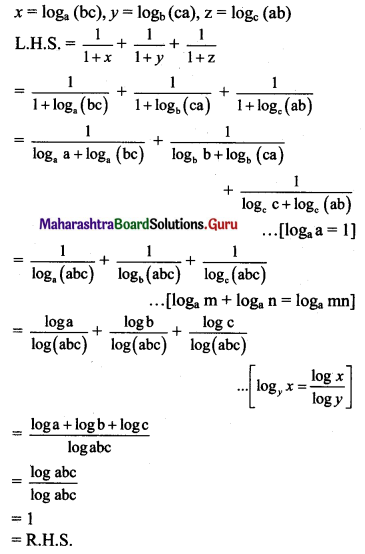Balbharti Maharashtra State Board 11th Maths Book Solutions Pdf Chapter 6 Functions Ex 6.1 Questions and Answers.

## Maharashtra State Board 11th Maths Solutions Chapter 6 Functions Ex 6.1

Question 1.
Check if the following relations are functions.
(a)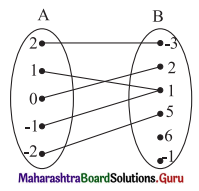Solution:
Yes.
Reason: Every element of set A has been assigned a unique element in set B.

(b)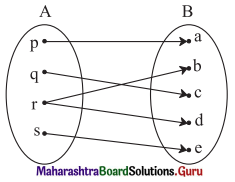Solution:
No.
Reason: An element of set A has been assigned more than one element from set B.

(c)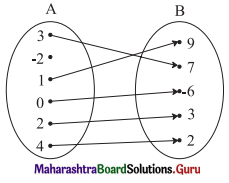Solution:
No.
Reason:
Not every element of set A has been assigned an image from set B.Question 2.
Which sets of ordered pairs represent functions from A = {1, 2, 3, 4} to B = {-1, 0, 1, 2, 3}? Justify.
(i) {(1, 0), (3, 3), (2, -1), (4, 1), (2, 2)}
(ii) {(1, 2), (2, -1), (3, 1), (4, 3)}
(iii) {(1, 3), (4, 1), (2, 2)}
(iv) {(1, 1), (2, 1), (3, 1), (4, 1)}
Solution:
(i) {(1, 0), (3, 3), (2, -1), (4, 1), (2, 2)} does not represent a function.
Reason: (2, -1), (2, 2), show that element 2 ∈ A has been assigned two images -1 and 2 from set B.

(ii) {(1, 2), (2, -1), (3, 1), (4, 3)} represents a function.
Reason: Every element of set A has been assigned a unique image in set B.

(iii) {(1, 3), (4, 1), (2, 2)} does not represent a function.
Reason:
3 ∈ A does not have an image in set B.

(iv) {(1, 1), (2, 1), (3, 1), (4, 1)} represents a function
Reason: Every element of set A has been assigned a unique image in set B.

Question 3.
Check if the relation given by the equation represents y as function of x.
(i) 2x + 3y = 12
(ii) x + y2 = 9
(iii) x2 – y = 25
(iv) 2y + 10 = 0
(v) 3x – 6 = 21
Solution:
(i) 2x + 3y = 12
∴ y = $$\frac{12-2 x}{3}$$
∴ For every value of x, there is a unique value of y.
∴ y is a function of x.

(ii) x + y2 = 9
∴ y2 = 9 – x
∴ y = ±$$\sqrt{9-x}$$
∴ For one value of x, there are two values of y.
∴ y is not a function of x.

(iii) x2 – y = 25
∴ y = x2 – 25
∴ For every value of x, there is a unique value of y.
∴ y is a function of x.

(iv) 2y + 10 = 0
∴ y = -5
∴ For every value of x, there is a unique value of y.
∴ y is a function of x.

(v) 3x – 6 = 21
∴ x = 9
∴ x = 9 represents a point on the X-axis.
There is no y involved in the equation.
So the given equation does not represent a function.Question 4.
If f(m) = m2 – 3m + 1, find
(i) f(0)
(ii) f(-3)
(iii) f($$\frac{1}{2}$$)
(iv) f(x + 1)
(v) f(-x)
(vi) $$\left(\frac{\mathbf{f}(2+h)-f(2)}{h}\right)$$, h ≠ 0.
Solution:
f(m) = m2 – 3m + 1
(i) f(0) = 02 – 3(0) + 1 = 1

(ii) f (-3) = (-3)2 – 3(-3) + 1
= 9 + 9 + 1
= 19

(iii) f($$\frac{1}{2}$$) = $$\left(\frac{1}{2}\right)^{2}-3\left(\frac{1}{2}\right)+1$$
= $$\frac{1}{4}-\frac{3}{2}+1$$
= $$\frac{1-6+4}{4}$$
= $$-\frac{1}{4}$$

(iv) f(x + 1) = (x + 1)2 – 3(x + 1) + 1
= x2 + 2x + 1 – 3x – 3 + 1
= x2 – x – 1

(v) f(-x) = (-x)2 – 3(-x) + 1 = x2 + 3x + 1

(vi) $$\left(\frac{\mathbf{f}(2+h)-f(2)}{h}\right)$$
= $$\frac{(2+h)^{2}-3(2+h)+1-\left(2^{2}-3(2)+1\right)}{h}$$
= $$\frac{\mathrm{h}^{2}+\mathrm{h}}{\mathrm{h}}$$
= h + 1

Question 5.
Find x, if g(x) = 0 where
(i) g(x) = $$\frac{5 x-6}{7}$$
(ii) g(x) = $$\frac{18-2 x^{2}}{7}$$
(iii) g(x) = 6x2 + x – 2
(iv) g(x) = x3 – 2x2 – 5x + 6
Solution:
(i) g(x) = $$\frac{5 x-6}{7}$$
g(x) = 0
∴ $$\frac{5 x-6}{7}$$ = 0
∴ x = $$\frac{6}{5}$$

(ii) g(x) = $$\frac{18-2 x^{2}}{7}$$
g(x) = 0
$$\frac{18-2 x^{2}}{7}$$ = 0
∴ 18 – 2x2 = 0
∴ x2 = 9
∴ x = ±3

(iii) g(x) = 6x2 + x – 2
g(x) = 0
∴ 6x2 + x – 2 = 0
∴ (2x – 1) (3x + 2) = 0
∴ 2x – 1 = 0 or 3x + 2 = 0
∴ x = $$\frac{1}{2}$$ or x = $$\frac{-2}{3}$$

(iv) g(x) = x3 – 2x2 – 5x + 6
= ( x- 1) (x2 – x – 6)
= (x – 1) (x + 2) (x – 3)
g(x) = 0
∴ (x – 1) (x + 2) (x – 3) = 0
∴ x – 1 = 0 or x + 2 = 0 or x – 3 = 0
∴ x = 1, -2, 3Question 6.
Find x, if f(x) = g(x) where
(i) f(x) = x4 + 2x2, g(x) = 11x2
(ii) f(x) = √x – 3, g(x) = 5 – x
Solution:
(i) f(x) = x4 + 2x2, g(x) = 11x2
f(x) = g(x)
∴ x4 + 2x2 = 11x2
∴ x4 – 9x2 = 0
∴ x2 (x2 – 9) = 0
∴ x2 = 0 or x2 – 9 = 0
∴ x = 0 or x2 = 9
∴ x = 0, ±3

(ii) f(x) = √x – 3, g(x) = 5 – x
f(x) = g(x)
∴ √x – 3 = 5 – x
∴ √x = 5 – x + 3
∴ √x = 8 – x
on squaring, we get
x = 64 + x2 – 16x
∴ x2 – 17x + 64 = 0
∴ x = $$\frac{17 \pm \sqrt{(-17)^{2}-4(64)}}{2}$$
∴ x = $$\frac{17 \pm \sqrt{289-256}}{2}$$
∴ x = $$\frac{17 \pm \sqrt{33}}{2}$$

Question 7.
If f(x) = $$\frac{a-x}{b-x}$$, f(2) is undefined, and f(3) = 5, find a and b.
Solution:
f(x) = $$\frac{a-x}{b-x}$$
Given that,
f(2) is undefined
b – 2 = 0
∴ b = 2 …..(i)
f(3) = 5
∴ $$\frac{a-3}{b-3}$$ = 5
∴ $$\frac{a-3}{2-3}$$ = 5 ….. [From (i)]
∴ a – 3 = -5
∴ a = -2
∴ a = -2, b = 2

Question 8.
Find the domain and range of the following functions.
(i) f(x) = 7x2 + 4x – 1
Solution:
f(x) = 7x2 + 4x – 1
f is defined for all x.
∴ Domain of f = R (i.e., the set of real numbers)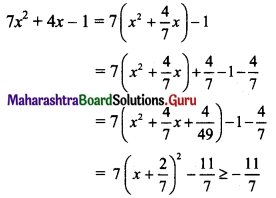∴ Range of f = [$$-\frac{11}{7}$$, ∞)

(ii) g(x) = $$\frac{x+4}{x-2}$$
Solution:
g(x) = $$\frac{x+4}{x-2}$$
Function g is defined everywhere except at x = 2.
∴ Domain of g = R – {2}
Let y = g(x) = $$\frac{x+4}{x-2}$$
∴ (x – 2) y = x + 4
∴ x(y – 1) = 4 + 2y
∴ For every y, we can find x, except for y = 1.
∴ y = 1 ∉ range of function g
∴ Range of g = R – {1}

(iii) h(x) = $$\frac{\sqrt{x+5}}{5+x}$$
Solution:
h(x) = $$\frac{\sqrt{x+5}}{5+x}=\frac{1}{\sqrt{x+5}}$$, x ≠ -5
For x = -5, function h is not defined.
∴ x + 5 > 0 for function h to be well defined.
∴ x > -5
∴ Domain of h = (-5, ∞)
Let y = $$\frac{1}{\sqrt{x+5}}$$
∴ y > 0
Range of h = (0, ∞) or R+

(iv) f(x) = $$\sqrt{x+1}$$
Solution:
f(x) = $$\sqrt{x+1}$$
f is defined for all real x and the values of f(x) ∈ R
∴ Domain of f = R, Range of f = R(v) f(x) = $$\sqrt{(x-2)(5-x)}$$
Solution:
f(x) = $$\sqrt{(x-2)(5-x)}$$
For f to be defined,
(x – 2)(5 – x) ≥ 0
∴ (x – 2)(x – 5) ≤ 0
∴ 2 ≤ x ≤ 5 ……[∵ The solution of (x – a) (x – b) ≤ 0 is a ≤ x ≤ b, for a < b]
∴ Domain of f = [2, 5]
(x – 2) (5 – x) = -x2 + 7x – 10
= $$-\left(x-\frac{7}{2}\right)^{2}+\frac{49}{4}-10$$
= $$\frac{9}{4}-\left(x-\frac{7}{2}\right)^{2} \leq \frac{9}{4}$$
∴ $$\sqrt{(x-2)(5-x)} \leq \sqrt{\frac{9}{4}} \leq \frac{3}{2}$$
Range of f = [0, $$\frac{3}{2}$$]

(vi) f(x) = $$\sqrt{\frac{x-3}{7-x}}$$
Solution:
f(x) = $$\sqrt{\frac{x-3}{7-x}}$$
For f to be defined,
$$\sqrt{\frac{x-3}{7-x}}$$ ≥ 0, 7 – x ≠ 0
∴ $$\sqrt{\frac{x-3}{7-x}}$$ ≤ 0 and x ≠ 7
∴ 3 ≤ x < 7
Let a < b, $$\frac{x-a}{x-b}$$ ≤ 0 ⇒ a ≤ x < b
∴ Domain of f = [3, 7)
f(x) ≥ 0 … [∵ The value of square root function is non-negative]
∴ Range of f = [0, ∞)

(vii) f(x) = $$\sqrt{16-x^{2}}$$
Solution:
f(x) = $$\sqrt{16-x^{2}}$$
For f to be defined,
16 – x2 ≥ 0
∴ x2 ≤ 16
∴ -4 ≤ x ≤ 4
∴ Domain of f = [-4, 4]
Clearly, f(x) ≥ 0 and the value of f(x) would be maximum when the quantity subtracted from 16 is minimum i.e. x = 0
∴ Maximum value of f(x) = √16 = 4
∴ Range of f = [0, 4]

Question 9.
Express the area A of a square as a function of its
(a) side s
(b) perimeter P
Solution:
(a) area (A) = s2
(b) perimeter (P) = 4s
∴ s = $$\frac{\mathrm{P}}{4}$$
Area (A) = s2 = $$\left(\frac{\mathrm{P}}{4}\right)^{2}$$
∴ A = $$\frac{\mathrm{P}^{2}}{16}$$Question 10.
Express the area A of a circle as a function of its
(ii) diameter d
(iii) circumference C
Solution:
(i) Area (A) = πr2

(ii) Diameter (d) = 2r
∴ r = $$\frac{\mathrm{d}}{2}$$
∴ Area (A) = πr2 = $$\frac{\pi \mathrm{d}^{2}}{4}$$

(iii) Circumference (C) = 2πr
∴ r = $$\frac{C}{2 \pi}$$
Area (A) = πr2 = $$\pi\left(\frac{\mathrm{C}}{2 \pi}\right)^{2}$$
∴ A = $$\frac{C^{2}}{4 \pi}$$

Question 11.
An open box is made from a square of cardboard of 30 cms side, by cutting squares of length x centimeters from each corner and folding the sides up. Express the volume of the box as a function of x. Also, find its domain.
Solution: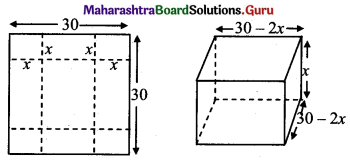Length of the box = 30 – 2x
Breadth of the box = 30 – 2x
Height of the box = x
Volume = (30 – 2x)2 x, x < 15, x ≠ 15, x > 0
= 4x(15 – x)2, x ≠ 15, x > 0
Domain (0, 15)

Question 12.
Let f be a subset of Z × Z defined by f = {(ab, a + b): a, b ∈ Z}. Is f a function from Z to Z? Justify?
Solution:
f = {(ab, a + b): a, b ∈ Z}
Let a = 1, b = 1. Then, ab = 1, a + b = 2
∴ (1, 2) ∈ f
Let a = -1, b = -1. Then, ab = 1, a + b = -2
∴ (1, -2) ∈ f
Since (1, 2) ∈ f and (1, -2) ∈ f,
f is not a function as element 1 does not have a unique image.

Question 13.
Check the injectivity and surjectivity of the following functions.
(i) f : N → N given by f(x) = x2
Solution:
f: N → N given by f(x) = x2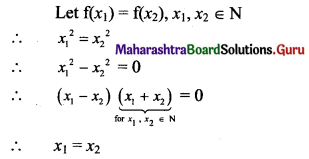∴ f is injective.
For every y = x2 ∈ N, there does not exist x ∈ N.
Example: 7 ∈ N (codomain) for which there is no x in domain N such that x2 = 7
∴ f is not surjective.

(ii) f : Z → Z given by f(x) = x2
Solution:
f: Z → Z given by f(x) = x2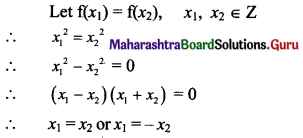∴ f is not injective.
(Example: f(-2) = 4 = f(2). So, -2, 2 have the same image. So, f is not injective.)
Since x2 ≥ 0,
f(x) ≥ 0
Therefore all negative integers of codomain are not images under f.
∴ f is not surjective.

(iii) f : R → R given by f(x) = x2
Solution:
f : R → R given by f(x) = x2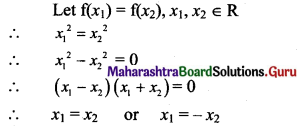∴ f is not injective.
f(x) = x2 ≥ 0
Therefore all negative integers of codomain are not images under f.
∴ f is not surjective.(iv) f : N → N given by f(x) = x3
Solution:
f: N → N given by f(x) = x3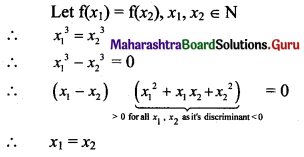∴ f is injective.
Numbers from codomain which are not cubes of natural numbers are not images under f.
∴ f is not surjective.

(v) f : R → R given by f(x) = x3
Solution:
f: R → R given by f(x) = x3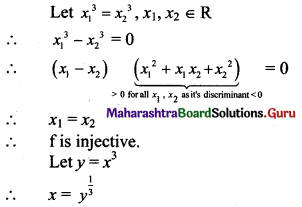∴ For every y ∈ R, there is some x ∈ R.
∴ f is surjective.

Question 14.
Show that if f : A → B and g : B → C are one-one, then gof is also one-one.
Solution:
f is a one-one function.
Let f(x1) = f(x2)
Then, x1 = x2 for all x1, x2 …..(i)
g is a one-one function.
Let g(y1) = g(y2)
Then, y1 = y2 for all y1, y2 …..(ii)
Let (gof) (x1) = (gof) (x2)
∴ g(f(x1)) = g(f(x2))
∴ g(y1) = g(y2),
where y1 = f(x1), y2 = f(x2) ∈ B
∴ y1 = y2 …..[From (ii)]
i.e., f(x1) = f(x2)
∴ x1 = x2 ….[From (i)]
∴ gof is one-one.

Question 15.
Show that if f : A → B and g : B → C are onto, then gof is also onto.
Solution:
Since g is surjective (onto),
there exists y ∈ B for every z ∈ C such that
g(y) = z …….(i)
Since f is surjective,
there exists x ∈ A for every y ∈ B such that
f(x) = y …….(ii)
(gof) x = g(f(x))
= g(y) ……[From (ii)]
= z …..[From(i)]
i.e., for every z ∈ C, there is x in A such that (gof) x = z
∴ gof is surjective (onto).

Question 16.
If f(x) = 3(4x+1), find f(-3).
Solution:
f(x) = 3(4x+1)
∴ f(-3) = 3(4-3+1)
= 3(4-2)
= $$\frac{3}{16}$$Question 17.
Express the following exponential equations in logarithmic form:
(i) 25 = 32
(ii) 540 = 1
(iii) 231 = 23
(iv) $$9^{\frac{3}{2}}$$ = 27
(v) 3-4 = $$\frac{1}{81}$$
(vi) 10-2 = 0.01
(vii) e2 = 7.3890
(viii) $$e^{\frac{1}{2}}$$ = 1.6487
(ix) e-x = 6
Solution: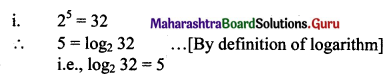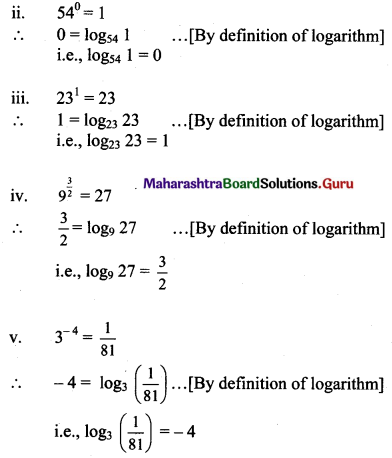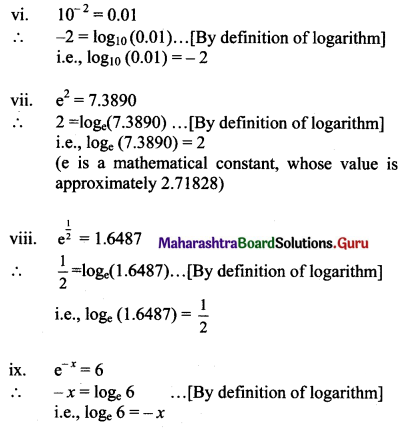Question 18.
Express the following logarithmic equations in exponential form:
(i) log2 64 = 6
(ii) $$\log _{5} \frac{1}{25}$$ = -2
(iii) log10 0.001 = -3
(iv) $$\log _{\frac{1}{2}}$$(8) = -3
(v) ln 1 = 0
(vi) ln e = 1
(vii) ln $$\frac{1}{2}$$ = -0.693
Solution:
(i) log2 64 = 6
∴ 64 = 26, i.e., 26 = 64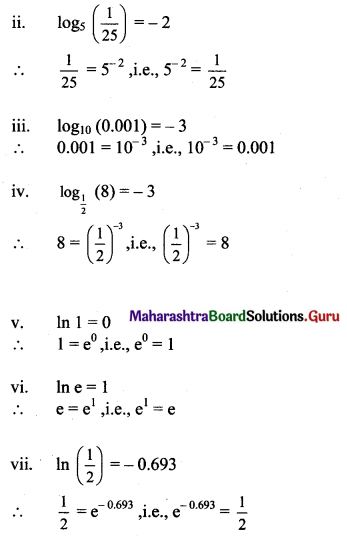Question 19.
Find the domain of
(i) f(x) = ln (x – 5)
(ii) f(x) = log10 (x2 – 5x + 6)
Solution:
(i) f(x) = ln (x – 5)
f is defined, when x – 5 > 0
∴ x > 5
∴ Domain of f = (5, ∞)

(ii) f(x) = log10 (x2 – 5x + 6)
x2 – 5x + 6 = (x – 2) (x – 3)
f is defined, when (x – 2) (x – 3) > 0
∴ x < 2 or x > 3
Solution of (x – a) (x – b) > 0 is x < a or x > b where a < b
∴ Domain of f = (-∞, 2) ∪ (3, ∞)Question 20.
Write the following expressions as sum or difference of logarithms:
(a) $$\log \left(\frac{p q}{r s}\right)$$
Solution: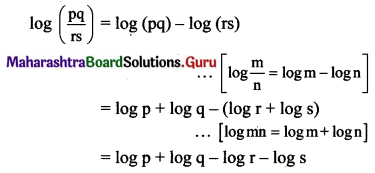(b) $$\log (\sqrt{x} \sqrt{y})$$
Solution: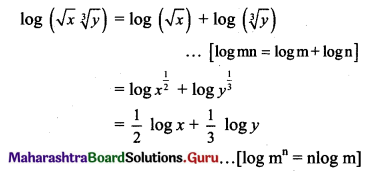(c) $$\ln \left(\frac{a^{3}(a-2)^{2}}{\sqrt{b^{2}+5}}\right)$$
Solution: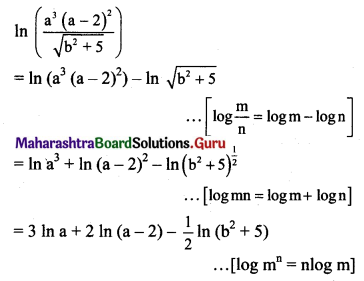(d) $$\ln \left[\frac{\sqrt{x-2}(2 x+1)^{4}}{(x+4) \sqrt{2 x+4}}\right]^{2}$$
Solution: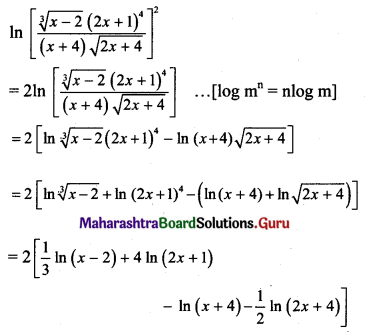Question 21.
Write the following expressions as a single logarithm.
(i) 5 log x + 7 log y – log z
Solution: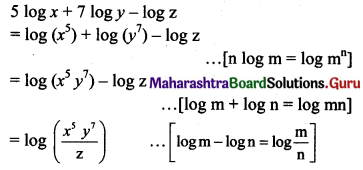(ii) $$\frac{1}{3}$$ log(x – 1) + $$\frac{1}{2}$$ log(x)
Solution: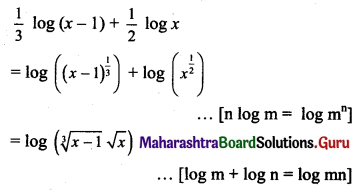(iii) ln (x + 2) + ln (x – 2) – 3 ln (x + 5)
Solution: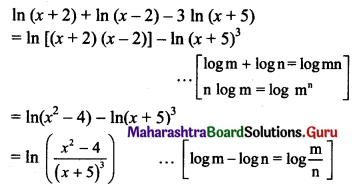Question 22.
Given that log 2 = a and log 3 = b, write log √96 terms of a and b.
Solution:
log 2 = a and log 3 = b
log √96 = $$\frac{1}{2}$$ log (96)
= $$\frac{1}{2}$$ log (25 x 3)
= $$\frac{1}{2}$$ (log 25 + log 3) …..[∵ log mn = log m + log n]
= $$\frac{1}{2}$$ (5 log 2 + log 3) ……[∵ log mn = n log m]
= $$\frac{5 a+b}{2}$$Question 23.
Prove that:
(a) $$b^{\log _{b} a}=a$$
Solution:
We have to prove that $$b^{\log _{b} a}=a$$
i.e., to prove that (logb a) (logb b) = logb a
(Taking log on both sides with base b)
L.H.S. = (logb a) (logb b)
= logb a …..[∵ logb b = 1]
= R.H.S.

(b) $$\log _{b^{m}} a=\frac{1}{m} \log _{b} a$$
Solution: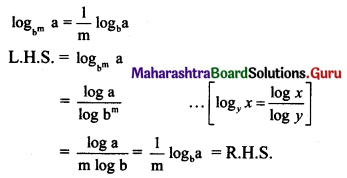(c) $$a^{\log _{c} b}=b^{\log _{c} a}$$
Solution: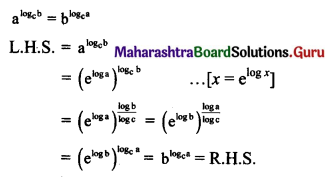Question 24.
If f(x) = ax2 – bx + 6 and f(2) = 3 and f(4) = 30, find a and b.
Solulion:
f(x) = ax2 – bx + 6
f(2) = 3
∴ a(2)2 – b(2) + 6 = 3
∴ 4a – 2b + 6 = 3
∴ 4a – 2b + 3 = 0 …..(i)
f(4) = 30
∴ a(4)2 – b(4) + 6 = 30
∴ 16a – 4b + 6 = 30
∴ 16a – 4b – 24 = 0 …..(ii)
By (ii) – 2 × (i), we get
8a – 30 = 0
∴ a = $$\frac{30}{8}=\frac{15}{4}$$
Substiting a = $$\frac{15}{4}$$ in (i), we get
4($$\frac{15}{4}$$) – 2b + 3 = 0
∴ 2b = 18
∴ b = 9
∴ a = $$\frac{15}{4}$$, b = 9

Question 25.
Solve for x:
(i) log 2 + log (x + 3) – log (3x – 5) = log 3
Solution:
log 2 + log (x + 3) – log (3x – 5) = log 3
∴ log 2(x + 3) – log(3x – 5) = log 3 …..[∵ log m + log n = log mn]
∴ log $$\frac{2(x+3)}{3 x-5}$$ = log 3 …..[∵ log m – log n = log $$\frac{m}{n}$$]
∴ $$\frac{2(x+3)}{3 x-5}$$ = 3
∴ 2x + 6 = 9x – 15
∴ 7x = 21
∴ x = 3

Check:
If x = 3 satisfies the given condition, then our answer is correct.
L.H.S. = log 2 + log (x + 3) – log (3x – 5)
= log 2 + log (3 + 3) – log (9 – 5)
= log 2 + log 6 – log 4
= log (2 × 6) – log 4
= log $$\frac{12}{4}$$
= log 3
= R.H.S.(ii) 2log10 x = 1 + $$\log _{10}\left(x+\frac{11}{10}\right)$$
Solution: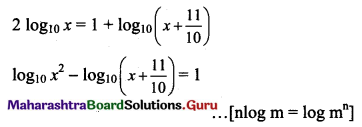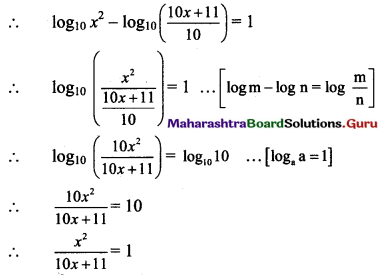∴ x2 = 10x + 11
∴ x2 – 10x – 11 = 0
∴ (x – 11)(x + 1) = 0
∴ x = 11 or x = -1
But log of a negative numbers does not exist
∴ x ≠ -1
∴ x = 11

(iii) log2 x + log4 x + log16 x = $$\frac{21}{4}$$
Solution: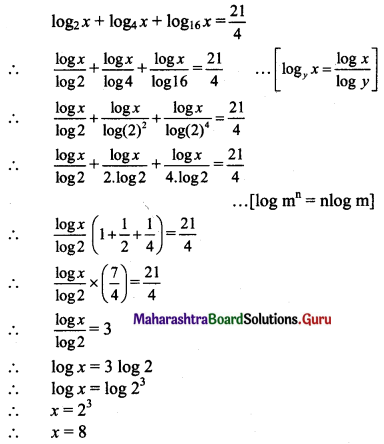(iv) x + log10 (1 + 2x) = x log10 5 + log10 6
Solution: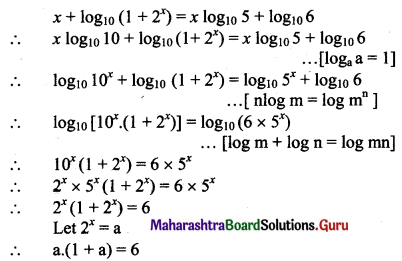∴ a + a2 = 6
∴ a2 + a – 6 = 0
∴ (a + 3)(a – 2) = 0
∴ a + 3 = 0 or a – 2 = 0
∴ a = -3 or a = 2
Since 2x = -3 is not possible,
2x = 2 = 21
∴ x = 1Question 26.
If log $$\left(\frac{x+y}{3}\right)$$ = $$\frac{1}{2}$$ log x + $$\frac{1}{2}$$ log y, show that $$\frac{x}{y}+\frac{y}{x}$$ = 7.
Solution: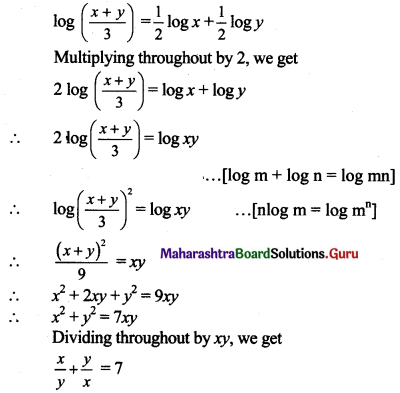Question 27.
If log$$\left(\frac{x-y}{4}\right)$$ = log√x + log√y, show that (x + y)2 = 20xy.
Solution: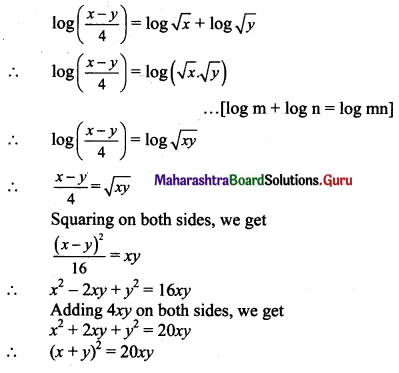Question 28.
If x = logabc, y = logb ca, z = logc ab, then prove that $$\frac{1}{1+x}+\frac{1}{1+y}+\frac{1}{1+z}$$ = 1.
Solution: